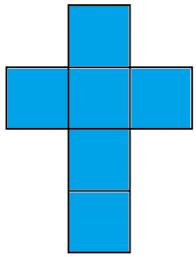# Cube 1-2-3

Calculate the volume and surface area of the cube ABCDEFGH if:
a) /AB/ = 4 cm
b) perimeter of wall ABCD is 22 cm
c) the sum of the lengths of all edges of the cube is 30 cm.

V1 =  64 cm3
S1 =  96 cm2
V2 =  166.375 cm3
S2 =  181.5 cm2
V3 =  15.625 cm3
S3 =  37.5 cm2

### Step-by-step explanation:Did you find an error or inaccuracy? Feel free to write us. Thank you!Tips to related online calculators
Tip: Our volume units converter will help you with the conversion of volume units.

## Related math problems and questions:

• CubeThe sum of all cube edges is 30cm. Find the surface area of the cube.
• Cube 7Calculate the volume of a cube, whose sum of the lengths of all edges is 276 cm.
• Prism XThe prism with the edges of the lengths x cm, 2x cm, and 3x cm has volume 20250 cm3. What is the area of the surface of the prism?
• Cube wallsFind the volume and surface area of the cube if the area of one wall is 40cm2.A quadrilateral pyramid, which has a rectangular base with dimensions of 24 cm, 13 cm. The height of the pyramid is 18cm. Calculate 1/the area of the base 2/casing area 3/pyramid surface 4/volume of the pyramid
• Cube wallThe perimeter of one cube wall is 120 meters. Calculate the surface area and the body diagonal of this cube.
• Cube edgesThe sum of the lengths of the cube edges is 42 cm. Calculate the surface of the cube.
• Sum of the edgesThe sum of the lengths of all edges of the cube is 72 cm. How many cm2 of colored paper are we going to use for sticking?Calculate the volume and surface area of a regular quadrangular prism 35 cm high and the base diagonal of 22 cm.
• Body diagonalCalculate the volume of a cuboid whose body diagonal u is equal to 6.1 cm. Rectangular base has dimensions of 3.2 cm and 2.4 cm
• Block or cuboidThe wall diagonals of the block have sizes of √29cm, √34cm, √13cm. Calculate the surface and volume of the block.
• Cube volumeThe cube has a surface of 384 cm2. Calculate its volume.
• Cuboid - ratiosThe sizes of the edges of the cuboid are in the ratio 2: 3: 5. The smallest wall has an area of 54 cm2. Calculate the surface area and volume of this cuboid.
• Surface of cubesPeter molded a cuboid 2 cm, 4cm, 9cm of plasticine. Then the plasticine split into two parts in a ratio 1:8. From each piece made a cube. In what ratio are the surfaces of these cubes?
• Wall diagonalCalculate the length of wall diagonal of the cube whose surface is 384 cm square.
• Edges or sidesCalculate the cube volume, if the sum of the lengths of all sides is 276 cm.
• Cube wallsFind the volume and the surface area of the cube if the area of one of its walls is 40 cm2.